# Competitiveness Evaluation of Tourist Attractions Based on Artificial Neural Network

Competitiveness Evaluation of Tourist Attractions Based on Artificial Neural Network
Management Department, Shijiazhuang University of Applied Technology, Shijiazhuang 050081, China

Center of International Cooperation and Exchange, Hebei Normal University, Shijiazhuang 050024, China

Corresponding Author Email:
malan@hebtu.edu.cn
Page:
623-630
|
DOI:
https://doi.org/10.18280/ria.340513
3 June 2020
|
Accepted:
17 September 2020
|
Published:
20 November 2020
| Citation

OPEN ACCESS

Abstract:

With the rapid development of tourism, tourists have become more aware of tourism level and quality. This triggers fierce competition between tourist attractions. To promote the core competitiveness of tourist attractions, this paper proposes a new evaluation model for the competitiveness of tourist attractions based on artificial neural network. First, a four-layer evaluation index system (EIS) was constructed for the competitiveness of tourist attractions, including detail elements, basic layer, core layer, and characterization layer. Next, all the evaluation indices were optimized through clustering by improved k-modes algorithm. Finally, a backpropagation neural network (BPNN) was established to evaluate the competitiveness of tourist attractions. Experimental results confirm the effectiveness of the proposed method. The research findings provide a reference for the application of artificial neural network (ANN) in other prediction fields.

Keywords:

backpropagation neural network (BPNN), k-modes algorithm, clustering and optimization, competitiveness of tourist attractions

1. Introduction

The consumption pattern of tourists has been changing constantly with the rapid development of tourism. Tourists have become more aware of tourism level and quality. This triggers fierce competition between tourist attractions. As a result, many scholars shift their attentions to the effective improvement of the core competitiveness of tourist attractions [1-4].

China is implementing supply-side reform of tourism, and upgrading the consumption pattern of tourists. In this context, the competitive advantages of tourist attractions are no longer limited to traditional elements like category, environment, and culture. Many new elements have emerged as the keys to improving the competitiveness of tourist attractions, including but not limited to dynamic management, marketing strategy, and tourist satisfaction. These elements reflect the degree of coordination between a tourist attraction, its managers, and tourists [5-9], which is positively correlated with the competitiveness of the attraction.

On the competitiveness of tourist attractions, the existing studies have covered all levels from country, province, city, attraction, enterprise, to market [10-12]. The main contents fall into three categories: the evaluation indices for competitiveness, the measurement and evaluation of competitiveness, and the improvement of competitiveness [13-15].

In terms of the evaluation indices for competitiveness, Salas-Olmedo et al.  constructed an EIS for the soft and hard competitiveness of provincial tourist attractions, which covers three aspects: the attractiveness of tourism resources, the support of the competitive environment, and the influence of the tourism resources market. Maeda et al.  conducted a systematic and in-depth study on the competitiveness of tourist attractions from eight aspects (economy, facilities, environment, talents, systems, services, performance, and openness, and created a reasonable EIS for urban tourism competitiveness.

In terms of the measurement and evaluation of competitiveness, Francalanci and Hussain  analyzed the international tourism competitiveness of four countries (China, the United States, Switzerland, and Thailand), modeled the international tourism competitiveness in four dimensions ( market demand, tourism product supply, environmental carrying capacity, and related industry support), and measured the international competitiveness of the tourism industry in these countries, with the aid of data envelopment analysis (DEA). Prayag et al.  combined the technique for order of preference by similarity to ideal solution (TOPSIS) and analytic hierarchy process (AHP) to comprehensively evaluate the international tourism competitiveness in 40 countries and regions, selected 30 evaluation indices from the performance, resources, and support of tourist attractions, and explained the evaluation process.

In terms of the improvement of competitiveness, Fahmi et al.  explored the tourism competitiveness system of cities in the Yangtze River Delta, and proposed multi-faceted strategies for enhancing the competitiveness of regional tourism cooperation. Under the background of informatization, Ibanez-Ruiz et al.  set up an EIS for the competitiveness of tourist attractions from the humanistic approach; Covering sense of belonging, sense of familiarity, sense of dependence, and sense of identity, the established EIS highlights the absolute relationship between tourist perception and national tourism competitiveness.

Despite their sheer number, the existing studies on the competitiveness of tourist attractions have rarely discussed in-depth strategies or models for competitiveness improvement through new methods or from new angles; the evaluation indices are highly correlated, and the evaluation methods and forms are far from diverse. New research on the competitiveness of tourist attractions should focus on the dynamic changes of the competitiveness, and innovate the evaluation method.

Artificial neural network (ANN) is a mature evaluation tools with excellence in data processing, self-adaptation, and self-learning. This paper puts forward a novel evaluation model for the competitiveness of tourist attractions based on the ANN. Firstly, a scientific EIS was established for the competitiveness of tourist attractions, which involves detail elements, basic layer, core layer, and characterization layer. Next, all the evaluation indices were optimized through clustering by improved k-modes algorithm. After that, an evaluation model for the competitiveness of tourist attractions was designed based on backpropagation neural network (BPNN). The effectiveness of our method was demonstrated through experiments.

2. EIS Construction

A scientific EIS is the basis for measuring and evaluating the competitiveness of tourist attractions. According to the provisions in the Detailed Rules for Rating Service Quality and Environmental Quality and the Detailed Rules for Rating Landscape Quality, the evaluation objective was determined based on the current situation of tourist attractions and innovation, and the traditional evaluation indices for the competitiveness of tourist attractions were merged, drawing on the classification criteria and methods for the quality of tourist attractions.

Referring to the relevant literature, a four-layer comprehensive, integral, scientific, and standard EIS was established for the competitiveness of tourist attractions. Involving detail elements, basic layer, core layer, and characterization layer, the EIS has four driving forces: potential, motivation, support, and attractiveness.

There are five primary indices in the EIS, including the experience of tour items, the experience of tourism services, the experience of tourism infrastructure, the experience of tourism management, and the experience of landscape atmosphere. The five primary indices are supported by 31 secondary indices.

## 1.jpg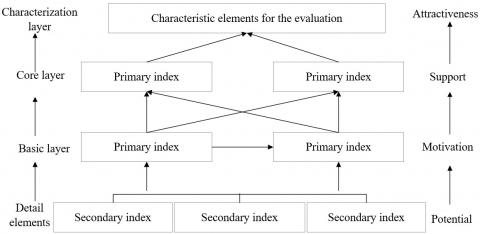Figure 1. The EIS for the competitiveness of tourist attractions

Table 1. The correlation levels between the primary indices

 Interval Type Level Interval Type Level [0, 0.1) Uncorrelated Extremely uncorrelated [0.5, 0.6) Correlated Nearly correlated [0.1, 0.2) Strongly uncorrelated [0.6, 0.7) Weakly correlated [0.2, 0.3) Moderately uncorrelated [0.7, 0.8) Moderately correlated [0.3, 0.4) Weakly uncorrelated [0.8, 0.9) Strongly correlated [0.4, 0.5) Nearly uncorrelated [0.9, 1] Extremely correlated

As shown in Figure 1, the entire hierarchical EIS is as follows:

Layer 1 (goal): C={competitiveness of tourist attractions};

Layer 2 (primary indices): C={C1, C2, C3, C4, C5}={experience of tour items, experience of tourism services, experience of tourism infrastructure, experience of tourism management, experience of landscape atmosphere};

Layer 3 (secondary indices);

C1={C11, C12, C13, C14, C15, C16, C17, C18, C19}={rich entertainment activities, reasonable food and beverage prices, unique food and beverages, good quality goods, unique goods, reasonable goods prices, reasonable accommodation prices, healthy and clean food and beverages, clean and tidy accommodation};

C2={C21, C22, C23, C24, C25, C26, C27}={patient and friendly attitude of service staff, high professionality of service staff, timely and efficiency handling of complaints, satisfactory handling of complaints, comprehensive and responsible services of tour guide, reasonable design of the travel route};

C3={C31, C32, C33, C34, C35}={clear and definite signages, convenient transportation, comfortable rest areas, sufficient and clean toilets, available facilities for special groups};

C4={C41, C42, C43, C44, C45, C46}={diverse and interesting performances, reasonably scheduled performances, coordination between tour items and atmosphere, matching between publicity and actual situation, reasonable ticket prices, proper control of the number of tourists};

C5={C51, C52, C53, C54}={clear theme, beautiful natural scenery, good security, strong historical and cultural atmosphere}.

This paper mainly analyzes the correlations among the five primary indices by the following correlation function:

$U=5 \sqrt{\frac{d_{1} d_{2} d_{3} d_{4} d_{5}}{d_{1}+d_{2}+d_{3}+d_{4}+d_{5}}}$  (1)

where, $U \in[0,1]$ is the value of the correlation function (if U=0, the correlations are the best, and the five primary indices are fully correlated and developing orderly; if U=1, the correlations are the worst, and the five primary indices are not

correlated and developing disorderly; the closer the U value is to 0, the poorer the correlations; the closer the U value is to 1, the better the correlations); d1-d5 are the levels of experience of tour items, experience of tourism services, experience of tourism infrastructure, experience of tourism management, experience of landscape atmosphere, respectively:

\begin{aligned} d_{1} &=\sum_{i=1}^{9} \lambda_{1 i} C_{1 i}, d_{2}=\sum_{i=1}^{7} \lambda_{2 i} C_{2 i}, d_{3}=\sum_{i=1}^{5} \lambda_{3 i} C_{3 i} \\ d_{4} &=\sum_{i=1}^{6} \lambda_{4 i} C_{4 i}, d_{5}=\sum_{i=1}^{4} \lambda_{5 i} C_{5 i} \end{aligned}  (2)

where, λ1i~λ5i are the weights of the five primary indices, respectively; C1i~C5i are the dimensionless values of the secondary indices under the five primary indices, respectively. Formula (1) must be corrected in case that the primary indices, which are highly correlated, have low correlation values:

$U^{*}=\sqrt{U\left(\alpha_{1} d_{1}+\alpha_{2} d_{2}+\alpha_{3} d_{3}+\alpha_{3} d_{3}+\alpha_{3} d_{3}\right)}$  (3)

where, U* is the degree of coordinated development of the contents in five primary indices; α1=α2=α3=α4=α5=0.2 are the coefficients of the contents in five primary indices (the coefficients are equal because the contents in different primary indices are of equal importance). Table 1 divides the correlations between the primary indices.

However, the dynamic change of the object in time is not fully considered. To solve the problem, the original model was improved by adding the adjustment coefficient of time change:

$U_{k j}^{*}=U_{k j} \times\left(1-\beta_{k}\right)^{j_{\max }-j}$  (4)

$\Delta d_{i y}=\frac{\left(A V_{k j}-A V_{k(j-1)}\right)}{A V_{k(j-1)}}, \beta_{k 1}=\frac{\sum_{j=j_{\min }}^{j_{\max }} \sum_{i=1}^{N_{k}} \lambda_{k i} C_{k i}}{j_{\max }-j_{\min }}$  (5)

where, AVkj is the mean of the k-th primary index of all tourist attractions in the 31 provincial administrative regions in China in year j; Δdkj is the growth rate of the k-th primary index in year j relative to the previous year; βk is the adjustment coefficient of the k-th primary index; Ukj is the correlation value of the k-th primary index in year j; Ukj* is the new correlation value of the k-th primary index in year j after being adjusted by the adjustment coefficient.

Figure 2 below displays the new correlation values of C1 experience of tour items given by ten experts.

## 2.jpg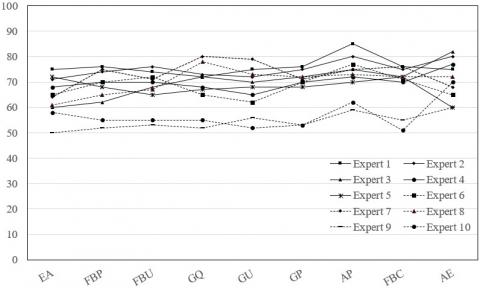Figure 2. The new correlation values of C1 experience of tour items

Note: EA is Entertainment Activities, FBP is Food and Beverage Prices, FBU is Food and Beverages Uniqueness, GQ is Goods Quality, GU is Goods Uniqueness, GP is Goods Prices, AP is Accommodation Prices, FBC is Food and Beverages Cleanliness, AE is Accommodation Environment.

3. Optimization Through K-Modes Clustering

Besides the accurate competitiveness evaluation of tourist attractions, this paper needs to fully consider the robustness and interpretability of the evaluation model. For this purpose, the traditional k-modes algorithm (Figure 3) was used to preliminarily cluster the data on the evaluation indices. The k-modes algorithm is suitable for local clustering and optimization of continuous numerical data. The objective function is the Euclidean distance from the data point to the prototype.

The traditional k-modes algorithm is easy to fall into local extreme points. Besides, the clustering classes often ignore the impact of the indices with low-frequency attributes.

## 3.jpg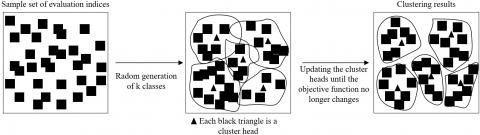Figure 3. The clustering process of traditional k-modes algorithm

This paper improves the k-modes clustering method to overcome the two defects. Firstly, the list of classification attributes of the evaluation indices was defined as CP＝(U, A, V, h), where U＝{u1, u2, …, un} is the set of index samples; A＝{a1, a2, …, am} is the set of index attributes; $V=\cup_{a} \in_{A} V_{a}$ is the union of attribute intervals; h is a function of C×AV (for any aÎA, and uÎU, there exists $h(u, \alpha) \in V_{a}$). In addition, it is assumed that Vaj＝{aj(1), aj(2), …, aj(nj)}, aj(l) is the l-th optional attribute value of attribute aj, and nj is the number of optional attributes.

Assuming that W＝[ω1, ω2, …, ωk]T, the p-th clustering of an index can be expressed as:

\begin{aligned} \omega_{p}=&\left[\omega_{p 11}, \omega_{p 12}, \ldots, \omega_{p 1 n_{1}}, \omega_{p 21}, \omega_{p 22}, \ldots,\right.\\ &\left.\omega_{p 2 n_{2}}, \ldots, \omega_{p m 1}, \omega_{p m 2}, \ldots, \omega_{p m n_{m}}\right]^{T} \end{aligned}  (6)

where, k is the number of clusters; 1≤pk; 1≤jm.

The above formula represents the weight vector considering the attribute values of all indices. Under the above assumptions, the distance between index sample ui and cluster head c can be calculated by:

$D I S\left(c_{p}, u_{i}\right)=\sum_{a \in A} \eta_{a}\left(c_{p}, u_{i}\right)$   (7)

where,

$\eta_{a}\left(c_{p}, u_{i}\right)=\left\{\begin{array}{l}1, h\left(c_{p}, a\right) \neq h\left(u_{i}, a\right) \\ 0, h\left(c_{p}, a\right)=h\left(u_{i}, a\right)\end{array}, 1 \leq p \leq k, 1 \leq i \leq n\right.$  (8)

The distance obtained by formula (7) characterizes the correlation difference between evaluation indices. Suppose V*Va1×Va2×…×Va|A|. The density of index sample ui belonging to V* can be expressed as:

$D E N\left(u_{i}\right)=\left(\frac{\left|\left\{v_{i} \in U \mid h\left(u_{i}, a\right)=h\left(v_{i}, a\right)\right\}\right|}{|U|}-1\right)$  (9)

The density obtained by formula (9) is the mean difference between an index sample and all the other index samples. The mode c of U that reflects the common feature of all indices can be calculated by:

$D E N(c)=\max _{u_{i} \in V^{*}} \operatorname{Dens}\left(u_{i}\right)$  (10)

The distance from the cluster head c directly bears on the possibility of index sample ui being a boundary point. Thus, the k samples with the farthest distance from c and the highest density were taken as the class samples for iterative selection. The first class samples can be obtained by:

$C S_{1}\left(u_{i}\right)=D E N\left(u_{i}\right)+D I S\left(u_{i}, c\right)$   (11)

The other class samples can be obtained through iteration:

$C S_{p+1}\left(u_{i}\right)=D E N\left(u_{i}\right)+\min _{j}^{p} D I S\left(v_{i}, c_{j}\right)$   (12)

Let ui be an index sample of class p, corresponding to candidate cluster head CCui＝{cc1, cc2, …cc|A|}, where cci is a mode in the set (12):

$M_{i}=\left\{v_{i} \in U \mid D I S\left(v_{i}, u_{i}\right) \leq i\right\}, 1 \leq i \leq|A|$   (13)

The final cluster head was selected from CCui. The selection principle is to choose the index sample with the highest inter-class distance, smallest intra-class distance, and highest density from vi in CCui. The cluster head of the first class can be obtained by:

Center $_{1}\left(v_{i}\right)=D E N\left(v_{i}\right)+D I S\left(v_{i}, c\right)-D I S\left(v_{i}, u_{i}\right)$  (14)

The cluster heads of the other classes can be obtained through iteration:

center$_{p_{+1}}\left(v_{i}\right)=D E N\left(v_{i}\right)+\min _{j=1}^{p} D I S\left(v_{i}, c_{j}\right)$$-D I S\left(v_{i}, u_{i}\right) (15) To consider the attribute values of all indices, the obtained cluster heads were transformed into the input weight cluster mode by the definition of mode W. If cluster head uijaj(s), the weight ωpjs equals 1; otherwise, ωpjs equals 0. Note that 1≤pk, 1≤jm, and 1≤snj. Thereby, the characteristic clustering class W1 was obtained for k classes. The objective function of the clustering can be defined as: O_{N}(H, W)=\sum_{p=1}^{k} \sum_{i=1}^{N} \theta_{p i} D I S_{N}\left(w_{p}, u_{i}\right) (16) where, H＝[θpi] is an k×N-dimensional 0-1 binary matrix. If index sample ui belongs to class p, θpi equals 1; otherwise, θpi equals 0. The distance between index samples can be calculated by: D I S_{N}\left(w_{p}, u_{i}\right)=\sum_{j=1}^{m} \rho_{a j}\left(w_{p}, u_{i}\right)$$=\sum_{j=1}^{m}\left(1-\omega_{p j t}\right)^{2}+\sum_{s=1, s \neq t}^{n_{j}} \omega_{p j s}^{2}$  (17)

If upjaj(t), then 1≤tnj. To minimize the objective function, H and W were iteratively updated by:

$\hat{\omega}_{p j t}=\frac{\left|n_{p j t}\right|}{\left|n_{p}\right|}$   (18)

$\hat{\theta}_{p i}=\left\{\begin{array}{ll}1, & D I S_{N}\left(\hat{\omega}_{p}, u_{i}\right) \leq D I S_{N}\left(\hat{\omega}_{g}, u_{i}\right), 1 \leq g \leq k \\ 0, & \text { Otherwise }\end{array}\right.$  (19)

where, |npjt| is the number of index samples in which the j-th attribute is of the value t in class p; |np| is the total number of index samples in class p. Since the updates of H and W satisfy the optimal Karush–Kuhn–Tucker (KKT) conditions, the objective function ON can converge. Moreover, the cluster heads obtained through iteration fully represent the features of the k clustering classes for the evaluation indices, which effectively prevents the falling into local extreme points. Hence, the optimal clustering results can be directly imported to the competitiveness evaluation model, making the evaluation more accurate. The clustering process of the improved algorithm is illustrated in Figure 4.

## 4.jpg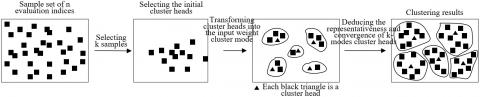Figure 4. The clustering process of improved k-modes algorithm

4. Model Construction

The BPNN learns through fastest gradient descent, and adjusts the thresholds and weights of the hidden layer through error backpropagation, thereby minimizing the sum of squares for error (SSE) between actual output and the desired output. To evaluate the competitiveness of tourist attractions, this paper constructs a BPNN in the following steps:

Step 1. Assign values $\in[-1,1]$ to the connection weights of each layer, configure the network structure, and determine the maximum number of learning and calculation accuracy.

Step 2. Randomly extract h samples from the n-dimensional input vector of evaluation indices, and express the desired output and characteristic input by:

$\left\{\begin{array}{l}e(h)=\left(e_{1}(h), e_{2}(h), \ldots, e_{m}(h)\right) \\ x(h)=\left(x_{1}(h), x_{2}(h), \ldots, x_{n}(h)\right)\end{array}\right.$  (20)

Step 3. Calculate the input HIp(H) and output HOp(H) of each hidden layer node backward layer by layer:

$H I_{p}(h)=\sum_{i=1}^{n} \omega_{i p} x_{i}(h)-\varepsilon_{p}, p=1,2, \ldots, m$   (21)

$H O_{p}(h)=g\left(H I_{p}(h)\right), p=1,2, \ldots, m$   (22)

Step 4. Based on the desired output and actual output of index samples, calculate the partial derivation of the error function relative to each output layer node:

$\frac{\partial e}{\partial o_{i}}=\frac{\partial\left(\frac{1}{2} \sum_{i=1}^{m}\left(e_{i}(h)-o_{i}(h)\right)^{2}\right)}{\partial o_{i}}$$=-\left(e_{i}(h)-o_{i}(h)\right) o_{i}(h) (23) By formula (23), compute the partial deviation of the error function relative to the connection weights between output layer and hidden layer: \frac{\partial e}{\partial \omega_{o p}}=\frac{\partial e}{\partial O_{i}} \cdot \frac{\partial O_{i}}{\partial \omega_{o p}}=\frac{\partial e}{\partial O_{i}} \cdot \frac{\partial\left(\sum_{p=1}^{q} \omega_{o p} H O_{p}(h)-\varepsilon_{p}\right)}{\partial \omega_{o p}}$$=\frac{\partial e}{\partial O_{i}} \cdot H O_{p}(h)$   (24)

Step 5. Based on the partial derivatives obtained above and the output of each hidden layer node, compute the partial derivative of the error function relative to each hidden layer node:

$\frac{\partial e}{\partial H I_{p}(h)}=\frac{\partial\left(\frac{1}{2} \sum_{i=1}^{m}\left(e_{i}(h)-o_{i}(h)\right)^{2}\right)}{\partial H O_{p}(h)} \cdot \frac{\partial H O_{p}(h)}{\partial H I_{p}(h)}$$=-\left(\sum_{p=1}^{m} \frac{\partial e}{\partial o_{i}} \cdot \omega_{p}\right) \cdot \frac{\partial H O_{p}(h)}{\partial H I_{p}(h)} (25) By formula (25), compute the partial deviation of the error function relative to the connection weights between hidden layer and input layer: \frac{\partial e}{\partial \omega_{i p}}=\frac{\partial e}{\partial H I_{p}(h)} \cdot \frac{\partial H I_{p}(h)}{\partial \omega_{i p}}$$=\frac{\partial e}{\partial H I_{p}(h)} \cdot \frac{\partial\left(\sum_{i=1}^{n} \omega_{i p} x_{i}(h)-\varepsilon_{p}\right)}{\partial \omega_{i p}}=\frac{\partial e}{\partial H I_{p}(h)} \cdot x_{i}(h)$   (26)

Step 6. Based on the connection weights between hidden layer and output layer and the partial deviation of the error function relative to each output layer node, adjust and update the weights through backpropagation of gradient, using the fastest gradient descent method:

$\left\{\begin{array}{c}\Delta \omega_{o p}(h)=-\eta \frac{\partial e}{\partial \omega_{o p}}=-\eta \frac{\partial e}{\partial o_{i}} \cdot H O_{p}(h) \\ \omega_{o p}^{t+1}=\omega_{o p}^{t}+\tau \frac{\partial e}{\partial o_{i}} \cdot H O_{p}(h)\end{array}\right.$  (27)

Step 7. Based on the connection weights between hidden layer and input layer and the partial deviation of the error function relative to these connection weights, adjust and update the weights through backpropagation of gradient, using the fastest gradient descent method:

$\left\{\begin{array}{c}\Delta \omega_{i p}(h)=-\eta \frac{\partial e}{\partial \omega_{i p}}=-\eta \frac{\partial e}{\partial H I_{p}(h)} \cdot x_{i}(k) \\ \omega_{i p}^{t+1}=\omega_{i p}^{t}+\tau \frac{\partial e}{\partial H I_{p}(h)} \cdot x_{i}(k)\end{array}\right.$  (28)

Step 8. Calculate the global error:

$E=\frac{1}{2 m} \sum_{k=1}^{m} \sum_{i=1}^{q}\left(d_{i}(k)-y_{o i}(k)\right)^{2}$  (29)

If the number of learning times reaches the preset maximum number or the global error meets the preset evaluation accuracy, terminate the iterative process; otherwise, return to Step 3 and execute the following steps until the network converges.

5. Experiments and Result Analysis

The contrastive experiments were conducted on a computer with Intel® Xeon® Processor E5620 (2.40 GHz) and 32G memory. The proposed algorithm was compared with k-nearest neighbors (KNN), traditional k-modes, k-means clustering (KMC), and fuzzy c-means (FCM)-KNN, in the Java language environment.

Table 2 compares the performance of KNN, traditional k-modes, and our algorithm in competitiveness evaluation of tourist attractions. It can be seen that our algorithm greatly outshined the other two methods in accuracy and recall.

The clustering results of all indices for the competitiveness of tourist attractions were summarized. Figure 5 presents the clustering results of primary index C2. Obviously, C21 belongs to class 1, C27 belongs to class 11, and C22-26 belong to class 5.

The proposed clustering algorithm was adopted to preliminarily cluster the evaluation indices. Figure 6 shows the relationship between minimum SSE and the number of classes K. It can be seen that the inflection point of the curve appeared at K=2 and 3; the number of index samples was correlated with the K value. The K value changes with the scale and type of the evaluation problem, and needs to be configured careful in actual application.

Table 2. The comparison between three clustering algorithms in competitiveness evaluation of tourist attractions

 Algorithm Cluster heads Number of samples Number of result samples Minimum SSE Accuracy Recall KNN (3,4,5,6) 1,546 775 1,564 0.9201 0.9213 (7,6,4,3) 656 (4,6,5,2) 583 Traditional k-modes (6,4,7,5) 1,546 526 947 0.9379 0.9476 (3,5,2,5) 673 (4,5,7,3) 711 Our algorithm (4,5,6,3) 1,546 803 703 0.9728 0.9841 (4,5,3,2) 523 (7,3,6,4) 476

## 5.jpg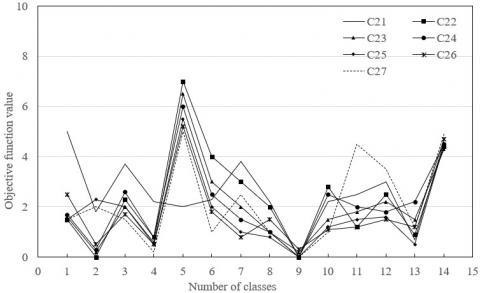Figure 5. The clustering results of primary index C2

## 6.jpg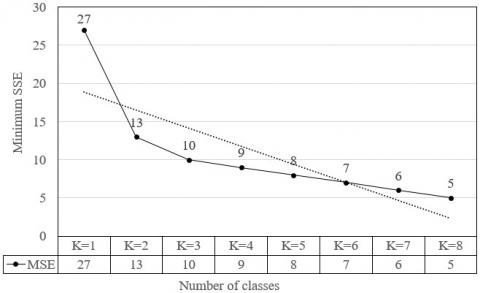Figure 6. The influence of number of classes on evaluation error

## 7a.jpg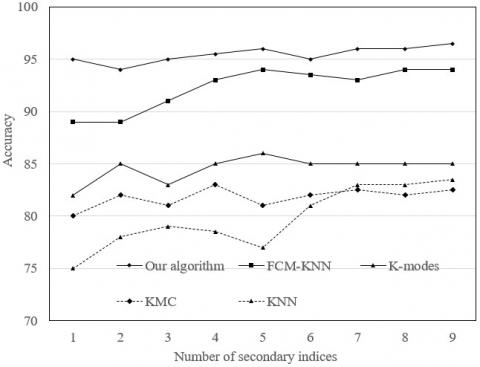(a) Secondary indices

## 7b.jpg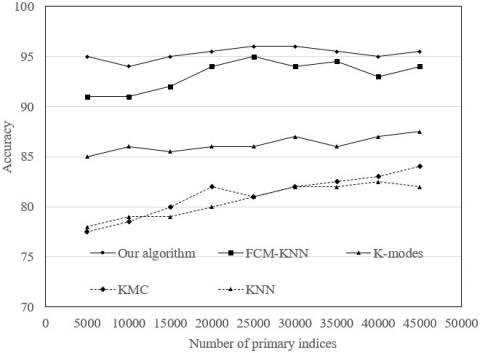(b) Primary indices

Figure 7. The influence of algorithm and number of index samples on accuracy

To verify the effect of different datasets with equal size on clustering accuracy, 31,224 test index samples were evenly divided into six groups for experimental analysis. Figure 7(a) presents the experimental results. It can be seen that KNN and traditional k-modes were low in accuracy, while our algorithm had a clear edge over the other four algorithms in evaluation accuracy. The main reasons are as follows:

The traditional K-modes computes the similarity between sample attributes based on distance to the boundary. The evaluation accuracy is greatly affected by the uncertain number of sample attributes. The KNN often mistakenly allocates lots of samples to the class of the largest group of index samples. The FCM-KNN generates the initial clusters randomly, which reduces the accuracy of the final classes. By contrast, the proposed algorithm can mitigate the negative effect of random initial clusters. In our algorithm, the initial cluster heads are selected based on both distance and density, preventing the falling into local extreme points. Through thorough consideration of the attribute values of all samples, the distance measure is effectively optimized. In this way, our method can adapt well to index data with class attributes, and realize high classification accuracy.

To verify the influence of sample number on clustering algorithms, eight groups of different number of primary index samples were adopted to compare the accuracies of different algorithms. As shown in Figure 7(b), our algorithm outperformed the other algorithms, regardless of the change in the number of index samples.

The next task is to verify the feasibility and effectiveness of the constructed BPNN. Under the initial training cycles of 3,000 and step length of 600, the output did not change significantly after 12,000 cycles. Hence, 12,000 was selected as the training cycles of the network.

The training period has a great impact on the evaluation accuracy and runtime of the neural network. If the period is too short, the network will have a poor data fitting effect; if the period is too long, the network could suffer from overfitting, i.e. the accuracy on test set is poorer than that on training set. Hence, this paper trains the network in 1,000 cycles, and observes the variation of evaluation accuracy with training cycles. As shown in Figure 8, the optimal number of training cycles for the BPNN is 200.

## 8.jpg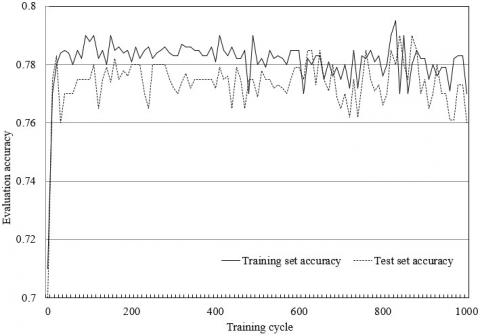Figure 8. The relationship between training cycle and evaluation accuracy

Figure 9 shows the distributions of the absolute error between the actual value and the corresponding output. It can be seen that the variance of the actual value was greater than that of the output, indicating that the fluctuation between the actual value and the mean value was larger than that between the output and the mean value. The main reason is the gap between the number of different primary indices, adding to the difficulty of network training and learning.

## 9.jpgFigure 9. The distributions of the absolute error between the actual value and the corresponding output

Figure 10 shows the relationship between the number of classes and the actual results of competitiveness evaluation. It can be seen that the actual values basically coincided with the desired outputs. This fully demonstrates the effectiveness of our BPNN.

## 10.jpg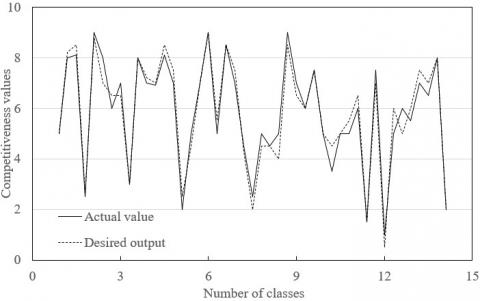Figure 10. The number of classes and the evaluation values

6. Conclusions

This paper introduces the BPNN to the competitiveness evaluation of tourist attractions, and puts forward a competitiveness evaluation model for tourist attractions. Firstly, a scientific EIS was designed for competitiveness evaluation of tourist attractions. The proposed EIS covers four layers, namely, detail elements, basic layer, core layer, and characterization layer, and has four driving forces: potential, motivation, support, and attractiveness. Then, all evaluation indices were optimized through the clustering by improved k-modes algorithm. Experimental results show that the proposed algorithm selects initial cluster heads based on both distance and density, preventing the falling into local extreme points; through thorough consideration of the attribute values of all samples, the distance measure is effectively optimized. Finally, the authors designed a BPNN for the competitiveness evaluation of tourist attractions. The experimental results show that the actual values basically coincided with the desired outputs, which fully demonstrates the effectiveness of our BPNN.

References

 Zhang, X., Luo, H., Chen, B., Guo, G. (2020). Multi-view visual Bayesian personalized ranking for restaurant recommendation. Applied intelligence, 50: 2901-2915. https://doi.org/10.1007/s10489-020-01703-6

 Liu, Y., Pan, S.L. (2015). Personalized travel recommendation technology based on friendship of LBSN. Computer Engineering and Applications, 51(8): 117-122.

 Park, D.H. (2017). The development of travel demand nowcasting model based on travelers' attention: focusing on web search traffic information. The Journal of Information Systems, 26(3): 171-185. https://doi.org/10.5859/KAIS.2017.26.3.171

 Maghrebi, M., Abbasi, A., Rashidi, T.H., Waller, S.T. (2015). Complementing travel diary surveys with twitter data: application of text mining techniques on activity location, type and time. 2015 IEEE 18th International Conference on Intelligent Transportation Systems, Las Palmas, pp. 208-213. https://doi.org/10.1109/ITSC.2015.43

 Abbasi, A., Rashidi, T.H., Maghrebi, M., Waller, S.T. (2015). Utilising location based social media in travel survey methods: Bringing Twitter data into the play. LBSN'15: Proceedings of the 8th ACM SIGSPATIAL International Workshop on Location-Based Social Networks, NY, US, pp. 1-9. https://doi.org/10.1145/2830657.2830660

 Pesonen, J., Lampi, M. (2016). Utilizing open data in tourism. ENTER 2016 Conference on Information and Communication Technologies in Tourism, Bilbao, Spain.

 Lim, K.H., Chan, J., Leckie, C., Karunasekera, S. (2018). Personalized trip recommendation for tourists based on user interests, points of interest visit durations and visit recency. Knowledge and Information Systems, 54(2): 375-406. https://doi.org/10.1007/s10115-017-1056-y

 Chong, W.H., Dai, B.T., Lim, E.P. (2015). Not all trips are equal: Analyzing foursquare check-ins of trips and city visitors. In Proceedings of the 2015 ACM on Conference on Online Social Networks, NY, US, pp. 173-184. https://doi.org/10.1145/2817946.2817958

 Padilla, J.J., Kavak, H., Lynch, C.J., Gore, R.J., Diallo, S.Y. (2018). Temporal and spatiotemporal investigation of tourist attraction visit sentiment on Twitter. PloS One, 13(6): e0198857. https://doi.org/10.1371/journal.pone.0198857

 Mariani, M., Baggio, R., Fuchs, M., Höepken, W. (2018). Business intelligence and big data in hospitality and tourism: A systematic literature review. International Journal of Contemporary Hospitality Management, 30(12): 3514-3554. https://doi.org/10.1108/IJCHM-07-2017-0461

 e Silva, F.B., Herrera, M.A.M., Rosina, K., Barranco, R. R., Freire, S., Schiavina, M. (2018). Analysing spatiotemporal patterns of tourism in Europe at high-resolution with conventional and big data sources. Tourism Management, 68: 101-115. https://doi.org/10.1016/j.tourman.2018.02.020

 Dwyer, L., Tomljenović, R., Čorak, S. (2017). Evolution of Destination Planning and Strategy. The Rise of Tourism in Croatia, Springer.

 Calheiros, A.C., Moro, S., Rita, P. (2017). Sentiment classification of consumer-generated online reviews using topic modeling. Journal of Hospitality Marketing & Management, 26(7): 675-693. https://doi.org/10.1080/19368623.2017.1310075

 Bunghez, C.L. (2016). The importance of tourism to a destination’s economy. Journal of Eastern Europe Research in Business & Economics, 143495. https://doi.org/10.5171/2016.143495

 Sabou, M., Onder, I., Brasoveanu, A.M., & Scharl, A. (2016). Towards cross-domain data analytics in tourism: a linked data based approach. Information Technology & Tourism, 16(1): 71-101. https://doi.org/10.1007/s40558-015-0049-5

 Salas-Olmedo, M.H., Moya-Gómez, B., García-Palomares, J.C., Gutiérrez, J. (2018). Tourists' digital footprint in cities: Comparing Big Data sources. Tourism Management, 66: 13-25. https://doi.org/10.1016/j.tourman.2017.11.001

 Maeda, T.N., Yoshida, M., Toriumi, F., Ohashi, H. (2018). Extraction of tourist destinations and comparative analysis of preferences between foreign tourists and domestic tourists on the basis of geotagged social media data. ISPRS International Journal of Geo-Information, 7(3): 99. https://doi.org/10.3390/ijgi7030099

 Francalanci, C., Hussain, A. (2016). Discovering social influencers with network visualization: evidence from the tourism domain. Information Technology & Tourism, 16(1): 103-125. https://doi.org/10.1007/s40558-015-0030-3

 Prayag, G., Hosany, S., Muskat, B., Del Chiappa, G. (2017). Understanding the relationships between tourists’ emotional experiences, perceived overall image, satisfaction, and intention to recommend. Journal of Travel Research, 56(1): 41-54. https://doi.org/10.1177/0047287515620567

 Fahmi, U., Ginting, N., Sitorus, R. (2018). Local communities and tourists’ perception towards to PLTD Apung sites as tsunami disaster tourism in Banda Aceh City. IOP Conference Series: Earth and Environmental Science, 126(1): 012177. https://doi.org/10.1088/1755-1315/126/1/012177

 Ibanez-Ruiz, J., Bustamante-Martinez, A., Sebastia, L., Onaindia, E. (2018). LinkedDBTour: A tool to retrieve linked open data about tourism attractions. 31st IBIMA Conference, Milan, Italy.